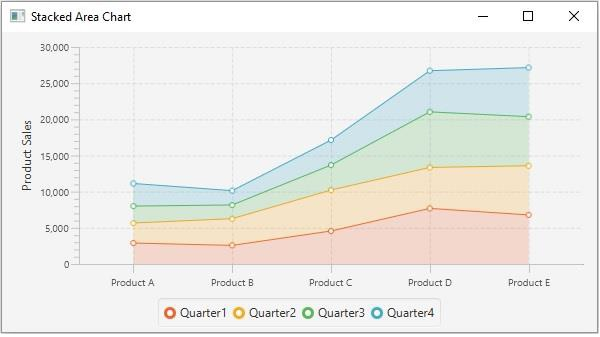# How to create a stacked area chart using JavaFX?

The area chart accepts a series of data points (x, y) as input values, connects them using a line, and maps the area between the obtained line and the axis.

StackedArea Chart is a variant of the Area Chart where the areas are stacked so that each series adjoins, but does not overlap the preceding series.

In JavaFX, you can create a stacked area chart by instantiating the javafx.scene.chart.StackedAreaChart class.

While instantiating this class you must pass the two objects of the Axis class representing the x and y-axis (as parameters of the constructor). Since the Axis class is abstract you need to pass objects of its concrete subclasses, NumberAxis (for numerical values) or, CategoryAxis (String values).

Once you create the axes you can set labels to them using the setLabel()method.

## Setting data

The XYChart.Series represents the series of data items. You can create a series of points for the line by instantiating this class. This class contains an observable list that holds all the points in the series.

The XYChart.Data represents a specific data point in the x-y plane. To create a point, you need to instantiate this class by passing the x and y values of the particular point.

## Example

import javafx.application.Application;
import javafx.scene.Scene;
import javafx.scene.chart.CategoryAxis;
import javafx.stage.Stage;
import javafx.scene.chart.NumberAxis;
import javafx.scene.chart.StackedAreaChart;
import javafx.scene.chart.XYChart;
import javafx.scene.layout.StackPane;
public class StackedAreaChartExample extends Application {
public void start(Stage stage) {
//Defining the axes
CategoryAxis xAxis = new CategoryAxis();
NumberAxis yAxis = new NumberAxis(0, 30000, 5000);
yAxis.setLabel("Product Sales");
//Creating the Area chart
StackedAreaChart<String, Number> areaChart = new StackedAreaChart(xAxis, yAxis);
//Prepare XYChart.Series objects by setting data
XYChart.Series series1 = new XYChart.Series();
series1.setName("Quarter1");
XYChart.Series series2 = new XYChart.Series();
series2.setName("Quarter2");
XYChart.Series series3 = new XYChart.Series();
series3.setName("Quarter3");
XYChart.Series series4 = new XYChart.Series();
series4.setName("Quarter4");
//Creating a Group object
StackPane pane = new StackPane(areaChart);
//Setting the scene
Scene scene = new Scene(pane, 595, 300);
stage.setTitle("Stacked Area Chart");
stage.setScene(scene);
stage.show();
}
public static void main(String args[]){
launch(args);
}
}

## Output